# SVG <path> elementGetting Started

cityseer is a collection of computational tools for fine-grained street-network and land-use analysis, useful for assessing the morphological precursors to vibrant neighbourhoods.

cityseer is underpinned by network-based methods that have been developed from the ground-up for micro-morphological urban analysis at the pedestrian scale, with the intention of providing contextually specific metrics for any given streetfront location. Importantly, cityseer computes metrics directly over the street network and offers distance-weighted variants. The combination of these strategies makes cityseer more contextually sensitive than methods otherwise based on crow-flies aggregation methods that do not take the network structure and its affect on pedestrian walking distances into account.

The use of python facilitates interaction with popular computational tools for network manipulation (e.g. networkX), geospatial data processing (e.g. shapely, etc.), Open Street Map workflows with OSMnx, and interaction with the numpy, geopandas (and momepy) stack of packages. The underlying algorithms are are implemented in numba JIT compiled code so that the methods can be applied to large decomposed networks. In-out convenience methods are provided for interfacing with networkX and graph cleaning tools aid the incorporation of messier network representations such as those derived from Open Street Map.

The github repository is available at github.com/benchmark-urbanism/cityseer-api. Associated papers introducing the package and demonstrating the forms of analysis that can be done with it are available at arXiv.

## SVG <path> elementInstallation

cityseer is a python package that can be installed with pip:

pip install cityseer

Code tests are run against python 3.9, though the code base will generally be compatible with Python 3.8+.

## SVG <path> elementNotebooks

The getting started guide on this page, and a growing collection of other examples, is available as an Jupyter Notebooks which can be accessed via the Examples page.

## SVG <path> elementQuickstart

cityseer revolves around networks (graphs). If you’re comfortable with numpy and abstract data handling, then the underlying data structures can be created and manipulated directly. However, it is generally more convenient to sketch the graph using NetworkX and to let cityseer take care of initialising and converting the graph for you.

# any networkX MultiGraph with 'x' and 'y' node attributes will do
# here we'll use the cityseer mock module to generate an example networkX graph
import networkx as nx
from cityseer.tools import mock, graphs, plot

G = mock.mock_graph()
print(nx.info(G))
# let's plot the network
plot.plot_nx(G, labels=True, node_size=80, dpi=200, figsize=(4, 4))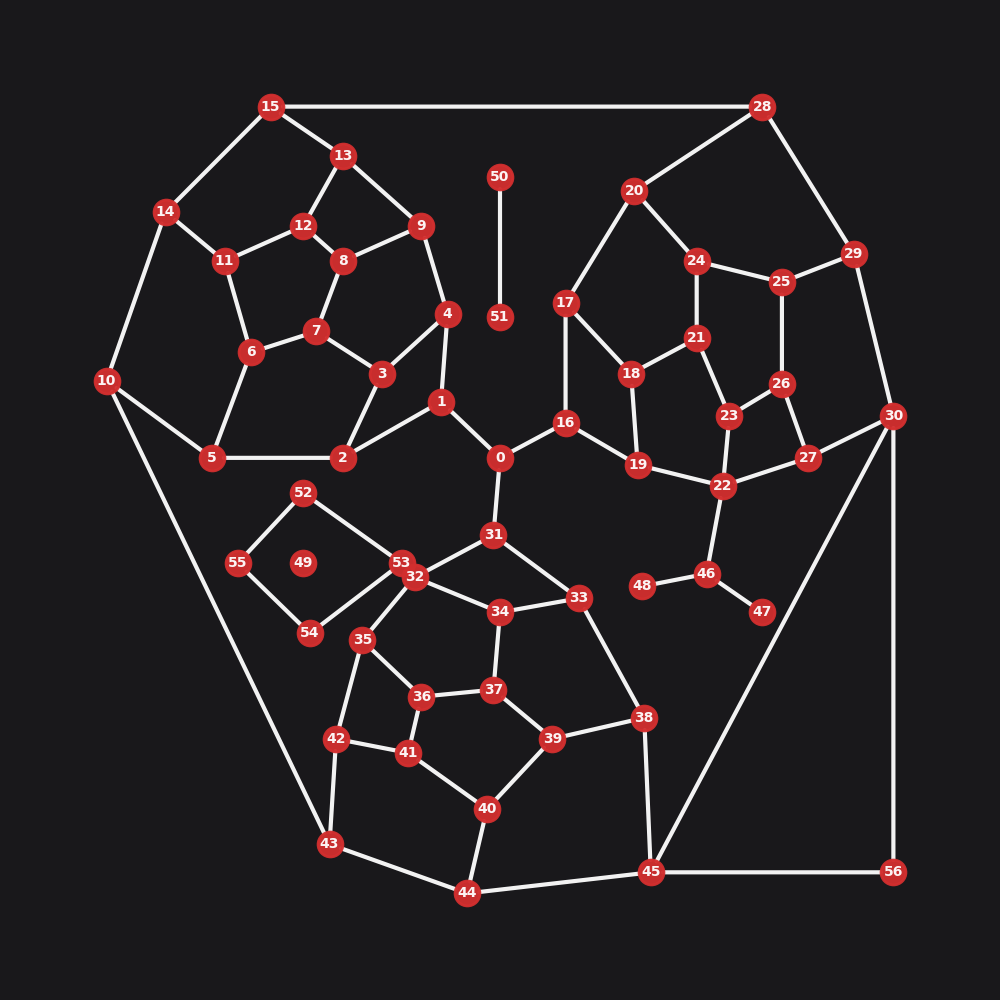An example graph.

## SVG <path> elementGraph Preparation

The tools.graphs module contains a collection of convenience functions for the preparation and conversion of networkX MultiGraphs, i.e. undirected graphs allowing for parallel edges. The tools.graphs module is designed to work with raw shapely Linestring geometries that have been assigned to the graph’s edge (link) geom attributes. The benefit to this approach is that the geometry of the network is decoupled from the topology: the topology is consequently free from distortions which would otherwise confound centrality and other metrics.

There are generally two scenarios when creating a street network graph:

1. In the ideal case, if you have access to a high-quality street network dataset – which keeps the topology of the network separate from the geometry of the streets – then you would construct the network based on the topology while assigning the roadway geometries to the respective edges spanning the nodes. OS Open Roads is a good example of this type of dataset. Assigning the geometries to an edge involves A) casting the geometry to a shapely LineString, and B) assigning this geometry to the respective edge by adding the LineString geometry as a geom attribute. e.g. G.add-edge(start_node, end_node, geom=a_linestring_geom).

2. In reality, most data-sources are not this refined and will represent roadway geometries by adding additional nodes to the network. For a variety of reasons, this is not ideal and you may want to follow the Graph Cleaning guide; in these cases, the graphs.nx_simple_geoms method can be used to generate the street geometries, after which several methods can be applied to clean and prepare the graph. For example, nx-wgs_to_utm aids coordinate conversions; nx_remove_dangling_nodes removes remove roadway stubs, nx_remove_filler_nodes strips-out filler nodes, and nx-consolidate-nodes assists in cleaning-up the network.

## SVG <path> elementExample

Here, we’ll walk through a high-level overview showing how to use cityseer. You can provide your own shapely geometries if available; else, you can auto-infer simple geometries from the start to end node of each network edge, which works well for graphs where nodes have been used to inscribe roadway geometries.

# use nx_simple_geoms to infer geoms for your edges
G = graphs.nx_simple_geoms(G)
plot.plot_nx(G, labels=True, node_size=80, plot_geoms=True, dpi=200, figsize=(4, 4))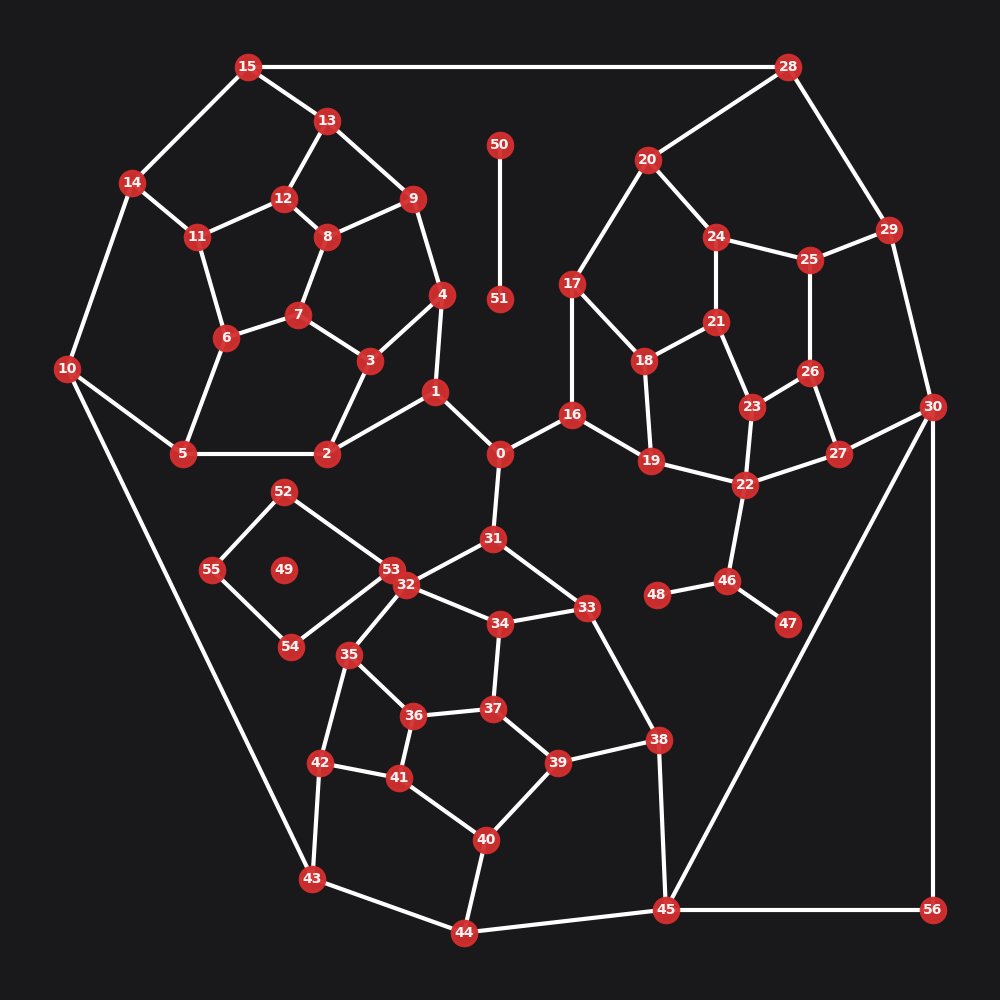A graph with inferred geometries. In this case the geometries are all exactly straight.

We have now inferred geometries for each edge, meaning that each edge now has an associated LineString geometry. Any further manipulation of the graph using the cityseer.graph module will retain and further manipulate these geometries in-place.

Once the geoms are readied, we can use tools such as nx_decompose for generating granular graph representations and nx_to_dual for casting a primal graph representation to its dual.

# this will (optionally) decompose the graph
G_decomp = graphs.nx_decompose(G, 50)
plot.plot_nx(G_decomp, plot_geoms=True, labels=False, dpi=200, figsize=(4, 4))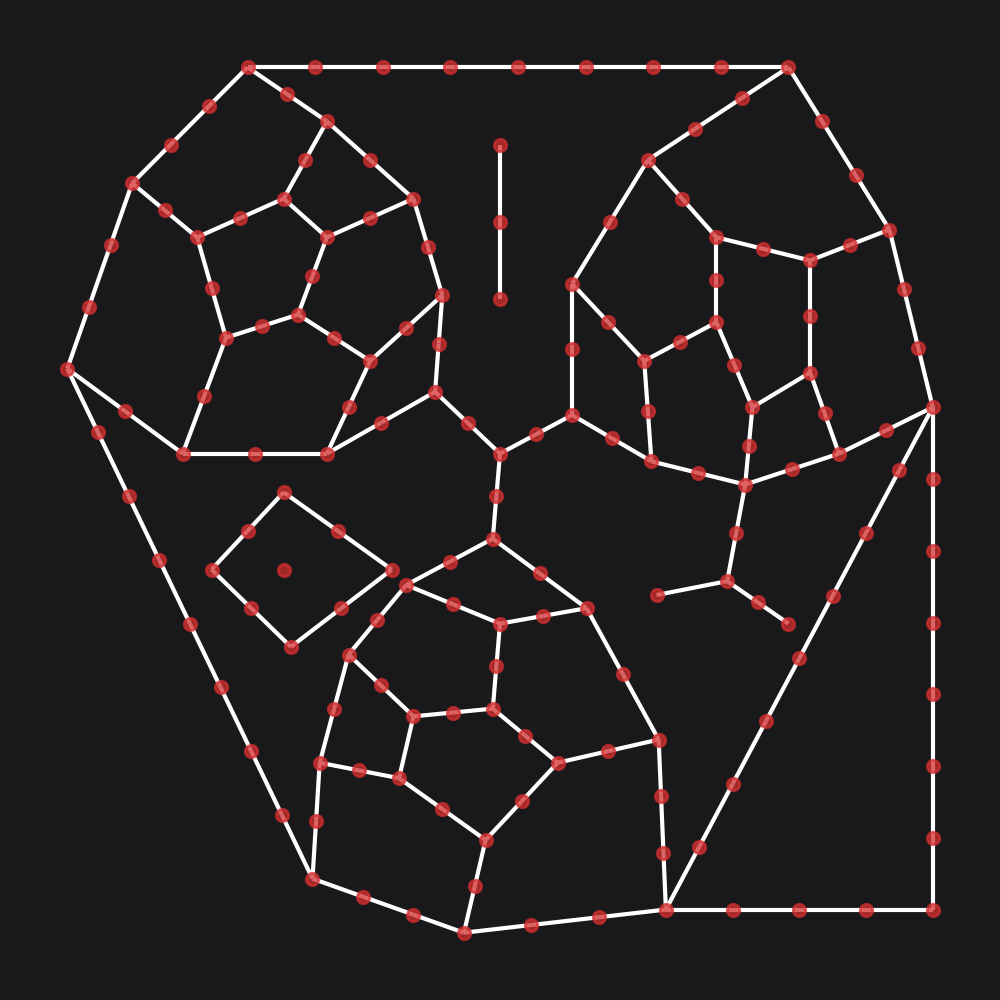A decomposed graph.

# this will (optionally) cast to a dual network
G_dual = graphs.nx_to_dual(G)
# here we are plotting the newly decomposed graph (blue) against the original graph (red)
plot.plot_nx_primal_or_dual(G, G_dual, plot_geoms=False, dpi=200, figsize=(4, 4))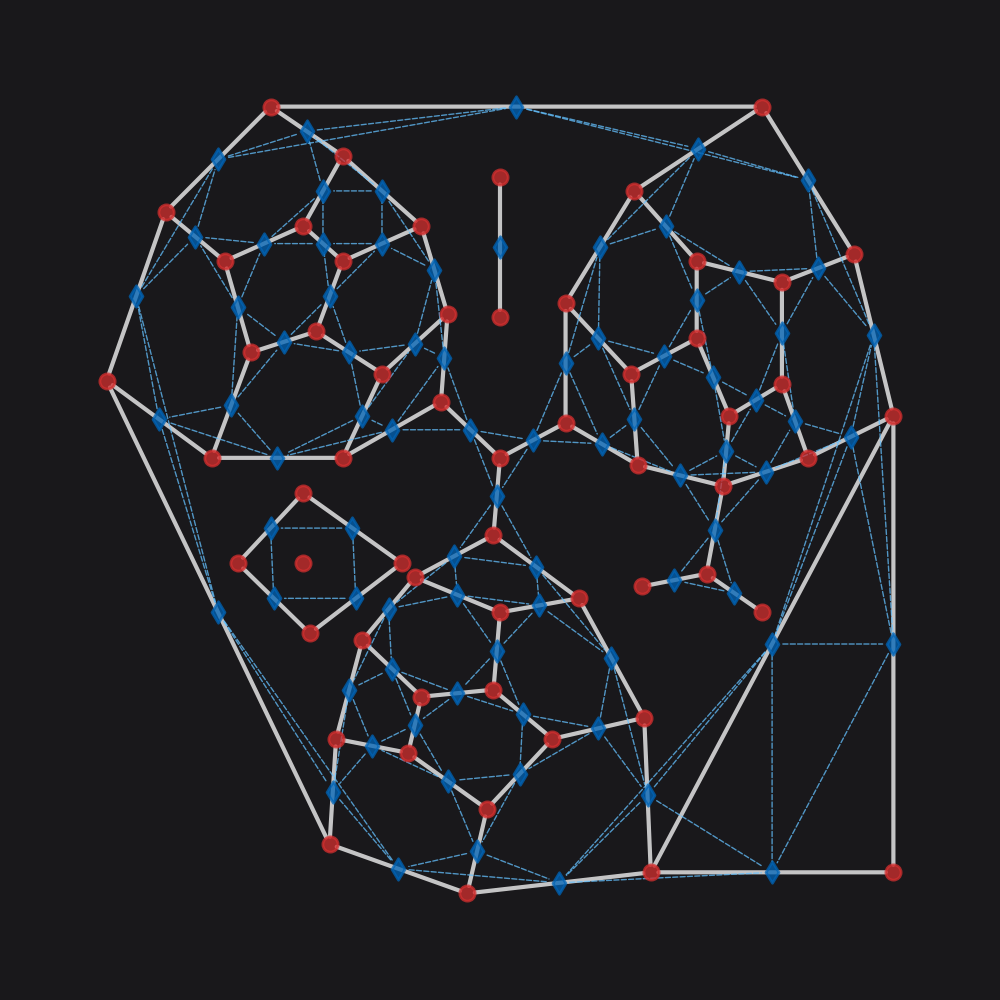A dual graph (blue) plotted against the primal source graph (red). In this case, the true geometry has not been plotted so that the dual graph is easily delineated from the primal graph.

## SVG <path> elementMetrics

After graph preparation and cleaning has been completed, the networkX graph can be transformed into data structures for efficiently computing centralities, land-use measures, or statistical aggregations.

Use network_structure_from_nx to convert a networkX graph into a GeoPandas GeoDataFrame and a structures.NetworkStructure, which is used by Cityseer for efficiently computing over the network.

### SVG <path> elementNetwork Centralities

The networks.node_centrality and networks.segment_centrality methods wrap underlying numba optimised functions that compute a range of centrality methods. All selected measures and distance thresholds are computed simultaneously to reduce the amount of time required for multi-variable and multi-scalar workflows. The results of the computations will be written to the GeoDataFrame.

from cityseer.metrics import networks

# create a Network layer from the networkX graph
# use a CRS EPSG code matching the projected coordinate reference system for your data
nodes_gdf, network_structure = graphs.network_structure_from_nx(G_decomp, crs=3395)
# the underlying method allows the computation of various centralities simultaneously, e.g.
nodes_gdf = networks.segment_centrality(
measures=["segment_harmonic", "segment_betweenness"],  # the form of centrality measures to compute - see docs
network_structure=network_structure,  # the network structure for which to compute the measures
nodes_gdf=nodes_gdf,  # the nodes GeoDataFrame, to which the results will be written
distances=[200, 400, 800, 1600],  # the distance thresholds for which to compute centralities
)
nodes_gdf.head()  # the results are now in the GeoDataFrame
# plot centrality
from matplotlib import colors

# custom colourmap
cmap = colors.LinearSegmentedColormap.from_list("cityseer", ["#64c1ff", "#d32f2f"])
# normalise the values
segment_harmonic_vals = nodes_gdf["cc_metric_segment_harmonic_800"]
segment_harmonic_vals = colors.Normalize()(segment_harmonic_vals)
# cast against the colour map
segment_harmonic_cols = cmap(segment_harmonic_vals)
# plot segment_harmonic
# cityseer's plot methods are used here and in tests for convenience
# that said, rather use plotting methods directly from networkX or GeoPandas where possible
plot.plot_nx(G_decomp, labels=False, node_colour=segment_harmonic_cols, dpi=200, figsize=(4, 4))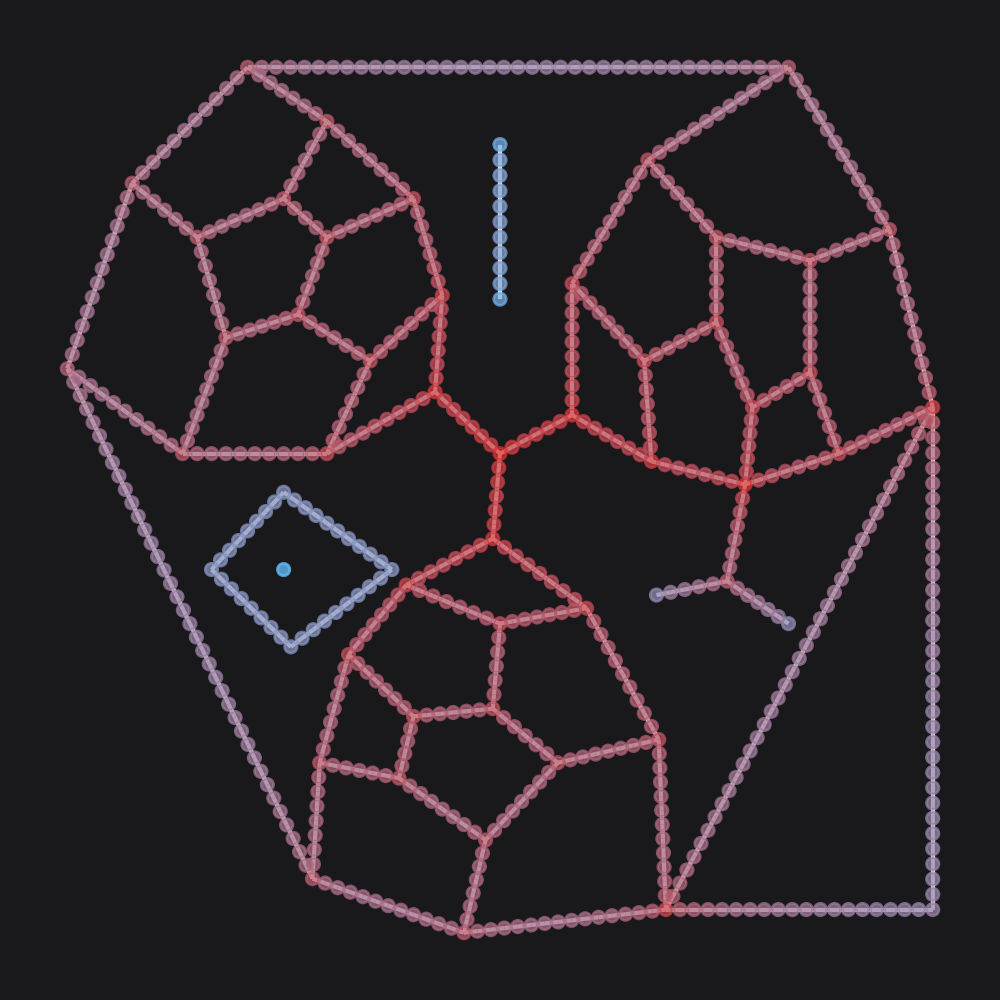800m segmentised harmonic centrality.

### SVG <path> elementLand-use and statistical measures

Landuse and statistical measures require a GeoPandas GeoDataFrame consisting of Point geometries. Columns representing categorical landuse information (“pub”, “shop”, “school”) can be passed to landuse methods, whereas columns representing numerical information can be used for statistical methods.

When computing these measures, cityseer will assign each data point to the two closest network nodes — one in either direction — based on the closest adjacent street edge. This enables cityseer to use dynamic spatial aggregation methods that more accurately describe distances from the perspective of pedestrians travelling over the network, and relative to the direction of approach.

layers.compute_landuses method is used for the calculation of mixed-use and land-use accessibility measures whereas layers.compute_stats can be used for statistical aggregations. As with the centrality methods, the measures are all computed simultaneously (and for all distances); however, simpler stand-alone methods are also available, including layers.hill_diversity, layers.hill_branch_wt_diversity, and layers.compute_accessibilities.

from cityseer.metrics import layers

# a mock data dictionary representing categorical landuse data
# here randomly generated letters represent fictitious landuse categories
data_gdf = mock.mock_landuse_categorical_data(G_decomp, random_seed=25)
data_gdf.head()
# example easy-wrapper method for computing mixed-uses
# this is a distance weighted form of hill diversity
nodes_gdf, data_gdf = layers.hill_branch_wt_diversity(
data_gdf,  # the source data
landuse_column_label="categorical_landuses",  # column in the dataframe which contains the landuse labels
nodes_gdf=nodes_gdf,  # nodes GeoDataFrame - the results are written here
network_structure=network_structure,  # measures will be computed relative to pedestrian distances over the network
distances=[200, 400, 800, 1600],  # distance thresholds for which you want to compute the measures
qs=[0, 1, 2],  # q parameter for hill mixed uses
)
print(nodes_gdf.columns)  # the GeoDataFrame will contain the results of the calculations
print(nodes_gdf["cc_metric_hill_branch_wt_q0_800"])  # which can be retrieved as needed
# for curiosity's sake - plot the assignments to see which edges the data points were assigned to
plot.plot_assignment(network_structure, G_decomp, data_gdf, dpi=200, figsize=(4, 4))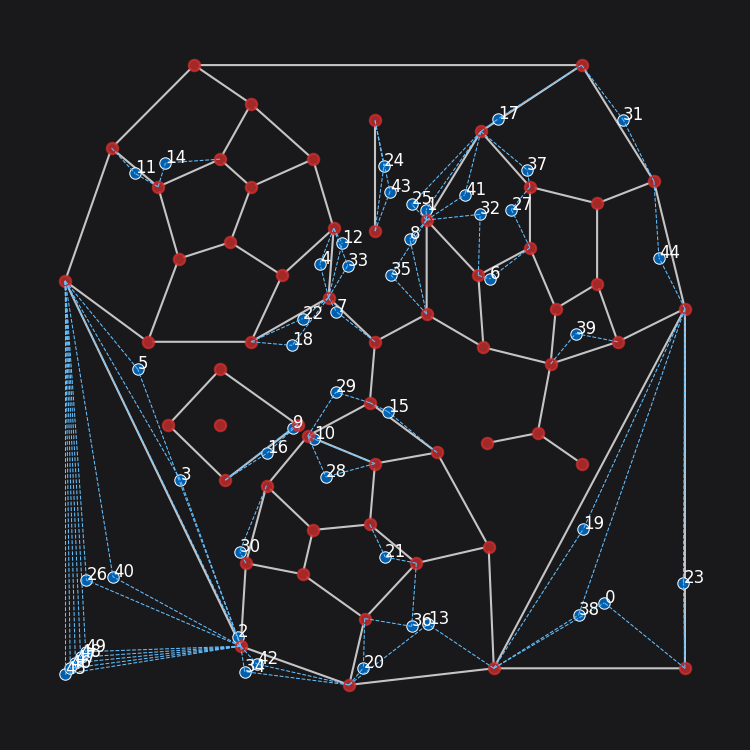Data points assigned to a Network Layer.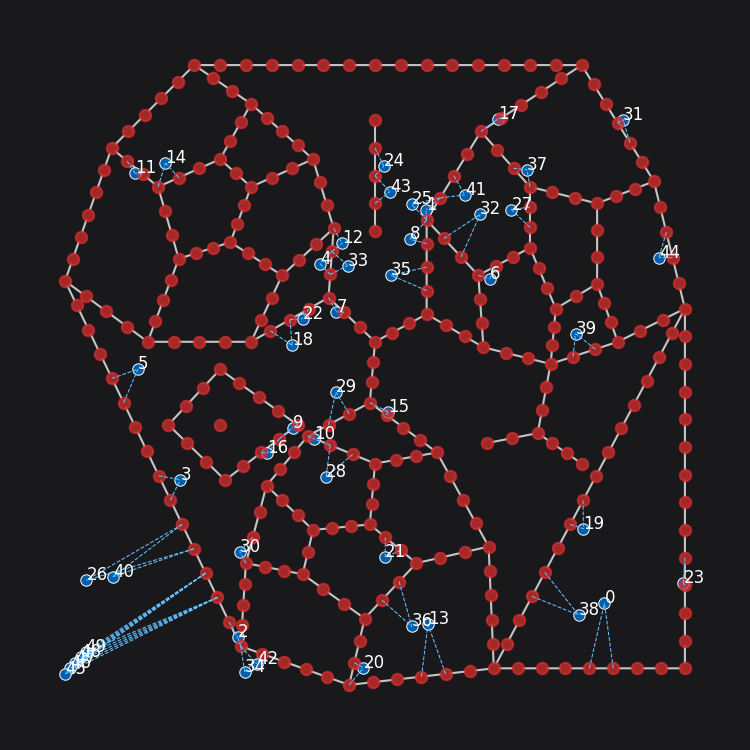Data assignment becomes more precise on a decomposed Network Layer.

# plot distance-weighted hill mixed uses
mixed_uses_vals = nodes_gdf["cc_metric_hill_branch_wt_q0_800"]
mixed_uses_vals = colors.Normalize()(mixed_uses_vals)
mixed_uses_cols = cmap(mixed_uses_vals)
plot.plot_assignment(
network_structure,
G_decomp,
data_gdf,
node_colour=mixed_uses_cols,
data_labels=data_gdf["categorical_landuses"].values,
dpi=200,
figsize=(4, 4),
)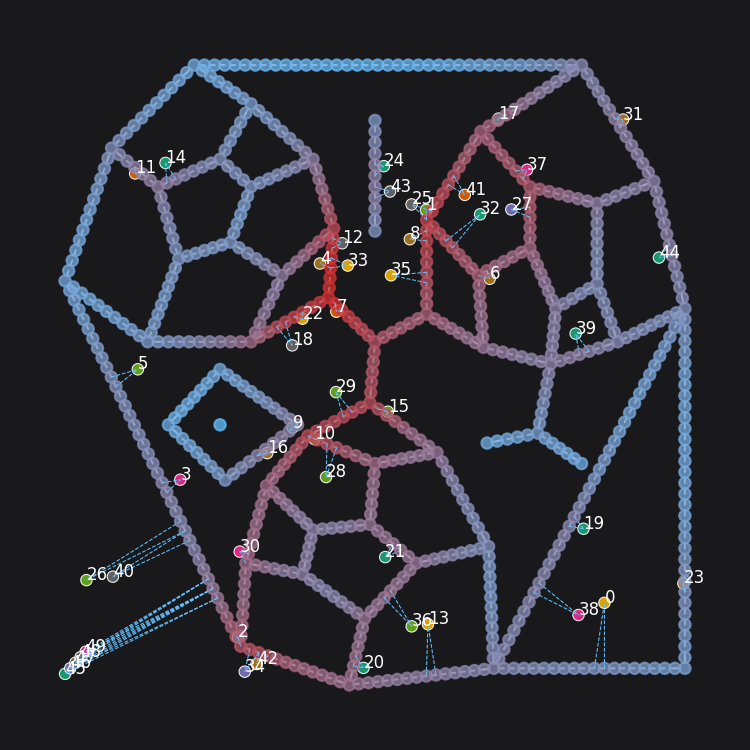400m distance-weighted mixed-uses.

# example easy-wrapper method for computing landuse accessibilities
nodes_gdf, data_gdf = layers.compute_accessibilities(
data_gdf,  # the source data
landuse_column_label="categorical_landuses",  # column in the dataframe which contains the landuse labels
accessibility_keys=["a", "b", "c"],  # the landuse categories for which to compute accessibilities
nodes_gdf=nodes_gdf,  # nodes GeoDataFrame - the results are written here
network_structure=network_structure,  # measures will be computed relative to pedestrian distances over the network
distances=[200, 400, 800, 1600],  # distance thresholds for which you want to compute the measures
)
# accessibilities are computed in both weighted and unweighted forms, e.g. for "a" and "b" landuse codes
print(nodes_gdf[["cc_metric_a_800_weighted", "cc_metric_b_1600_non_weighted"]])  # and can be retrieved as needed

Aggregations can likewise be computed for numerical data. Let’s generate some mock numerical data:

numerical_data_gdf = mock.mock_numerical_data(G_decomp, num_arrs=3)

# example easy-wrapper method for computing landuse accessibilities
nodes_gdf, numerical_data_gdf = layers.compute_stats(
numerical_data_gdf,  # the source data
stats_column_labels=["mock_numerical_1", "mock_numerical_2"],  # numerical columns to compute stats for
nodes_gdf=nodes_gdf,  # nodes GeoDataFrame - the results are written here
network_structure=network_structure,  # measures will be computed relative to pedestrian distances over the network
distances=[800, 1600],  # distance thresholds for which you want to compute the measures
)
# statistical aggregations are calculated for each requested column, and in the following forms:
# max, min, sum, sum_weighted, mean, mean_weighted, variance, variance_weighted

print(nodes_gdf["cc_metric_mock_numerical_2_max_800"])
print(nodes_gdf["cc_metric_mock_numerical_1_mean_weighted_800"])

The landuse metrics and statistical aggregations are computed over the street network relative to the network, with results written to each node. The mixed-use, accessibility, and statistical aggregations can therefore be compared directly to centrality computations from the same locations, and can be correlated or otherwise compared.

Data derived from metrics can be converted back into a NetworkX graph using the nx_from_network_structure method, or can otherwise be used to overlay computed metrics onto the original graph by providing the nx_multigraph parameter.

nx_multigraph_round_trip = graphs.nx_from_network_structure(
nodes_gdf=nodes_gdf, network_structure=network_structure, nx_multigraph=G_decomp
)
nx_multigraph_round_trip.nodes